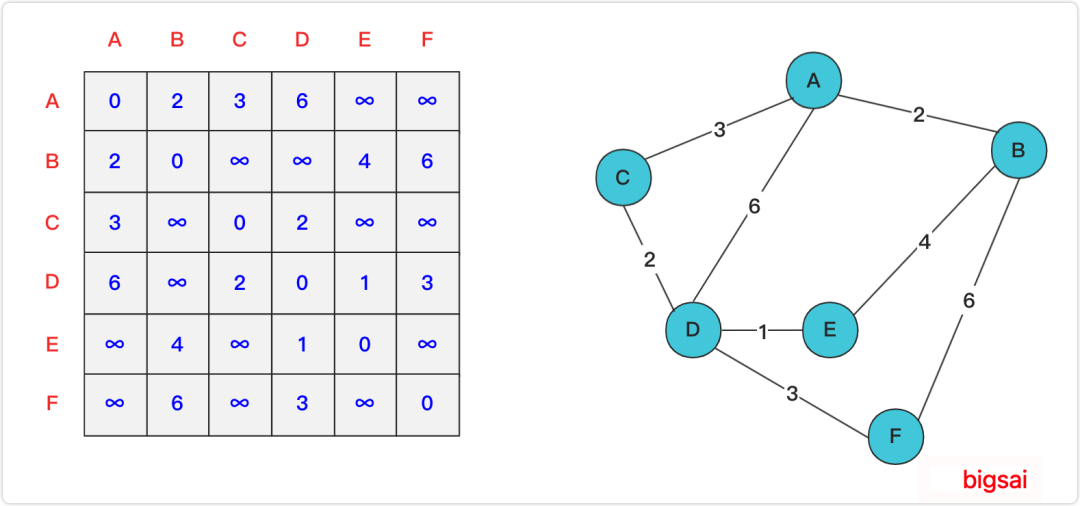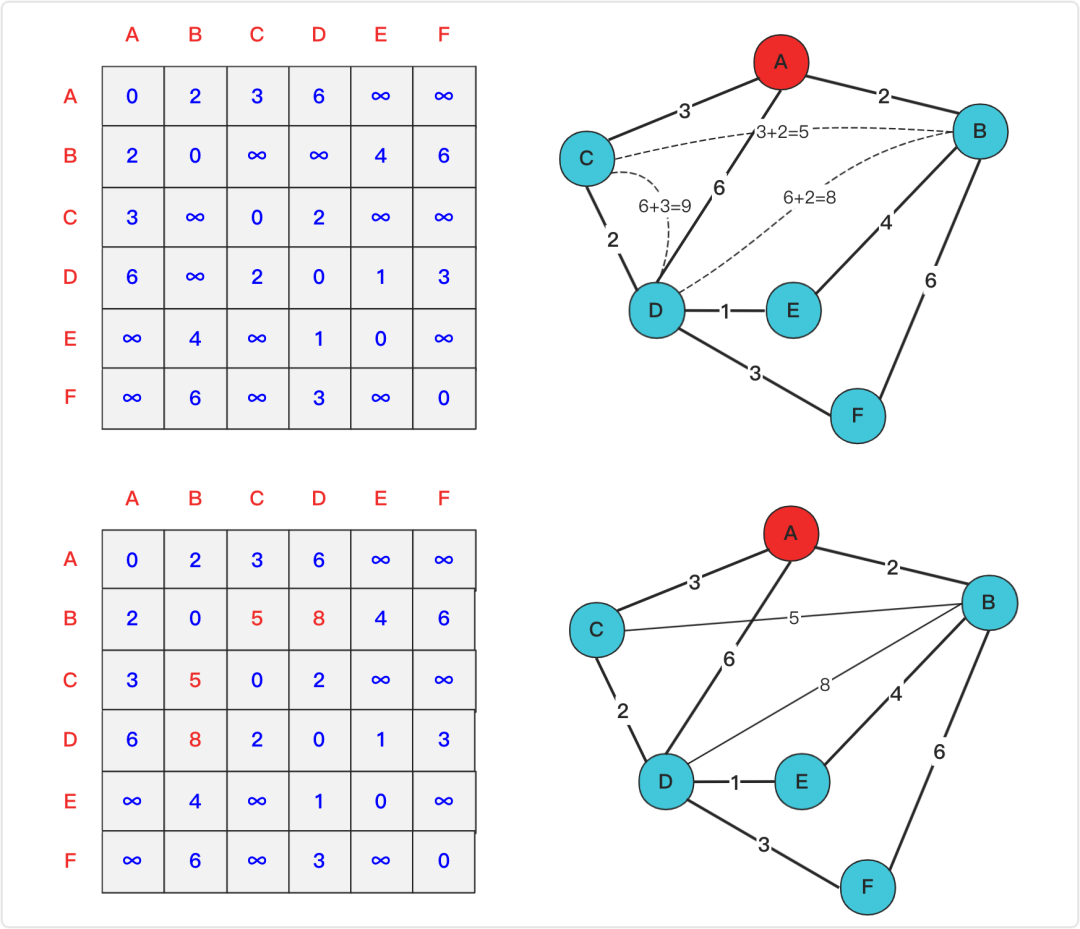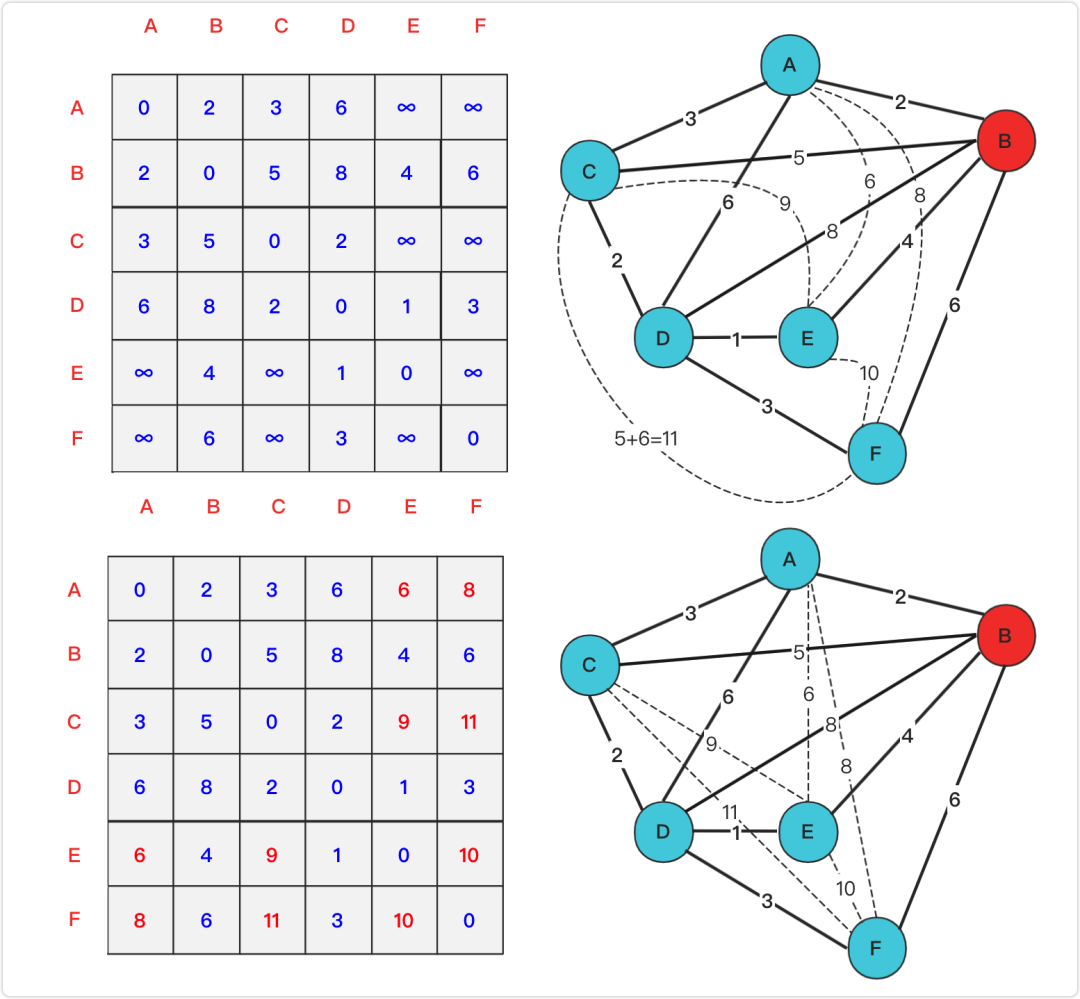# Floyd是咋求图的最短路径?（一）

joytrian

0收藏

Floyd算法又称为插点法，是一种利用动态规划的思想寻找给定的加权图中多源点之间最短路径的算法，与Dijkstra算法类似。该算法名称以创始人之一、1978年图灵奖获得者、斯坦福大学计算机科学系教授罗伯特·弗洛伊德命名。

1 .邻接矩阵(二维数组)dist储存路径，数组中的值开始表示点点之间初始直接路径，最终是点点之间的最小路径，有两点需要注意的，第一是如果没有直接相连的两点那么默认为一个很大的值(不要因为计算溢出成负数)，第二是自己和自己的距离要为0。

2 .从第1个到第n个点依次加入松弛计算，每个点加入进行试探枚举是否有路径长度被更改(自己能否更新路径)。顺序加入(k枚举)松弛的点时候，需要遍历图中每一个点对(i,j双重循环)，判断每一个点对距离是否因为加入的点而发生最小距离变化，如果发生改变(变小)，那么两点(i,j)距离就更改。

2 .重复上述直到最后插点试探完成。

``dp[i][j]=min(dp[i][j],dp[i][k]+dp[k][j])``AF1=AB+BF=6+2=8 < AF0(∞) 进行更新

AE1=AB+BE=2+4=6 < AE0(∞) 进行更新

CE1=CB+BE=5+5=9 < CE0(∞) 进行更新

CF1=CB+BF=5+6=11<CF0(∞) 进行更新

EF1=EB+BF=4+6=10<EF0(∞) 进行更新

AC1=AB+BC=2+5=7>AC0(3) 不更新

……社区精华内容